A Definitions, derivations and tricks

## Appendix ADefinitions, derivations and tricks

Paleomagnetism is famous for its use of a large number of incomprehensible acronyms. Here we have them gathered together along with definitions and the Section numbers where they are explained in more detail. You will find here a table of physical constants and paleomagnetic parameters used in the text as well as a table listing common statistics used in paleomagnetism. After the tables, there are a few sections with useful mathematical tricks.

### A.1 Definitions

 Table A.1: Acronyms in paleomagnetism. Acronym Definition: Section # AMS Anisotropy of magnetic susceptibility: Section 13.1 APWP Apparent polar wander path: Section 16.2 AF Alternating field demagnetization: Section 9.4 ARM Anhysteretic remanent magnetization: Section 7.10 ChRM Characteristic remanent magnetization: Section 9.5 CNS Cretaceous Normal Superchron: Section 15.1 CRM Chemical remanent magnetization: Section 7.5 DGRF Definitive geomagnetic reference field: Section 2.2 DRM Detrital remanent magnetization: Section 7.6 E/I Elongation/inclination correction method: Section 16.4 FC Field cooled: Section 8.8.4 GAD Geocentric axial dipole: Section 2.3 GHA Greenwich hour angle: Appendix A.3.8 GPTS Geomagnetic polarity time scale: Chapter 15 GRM Gyroremanent magnetization: Section 7.10 IGRF International geomagnetic reference field: Section 2.2 IZZI Infield-zero field/ zero field-infield paleointensity protocol: Section 10.1.1 IRM Isothermal remanent magnetization: Sections 5.2.1 and s7.7 MD Multi-domain: Chapter 4 MDF Median destructive field: Section 8.2 MDT Median destructive temperature: Section 8.2 NRM Natural remanent magnetization: Chapter 7 pARM Partial anhysteretic remanence: Section 7.10 pDRM Post-depositional detrital remanent magnetization: Section 7.6 PSD Pseudo-single domain: Chapter 4 PSV Paleosecular variation of the geomagnetic field: Section 14.1 pTRM Partial thermal remanence: Section 7.4 sIRM Saturation IRM: See Mr SD Single domain: Chapter 4 SP Superparamagnetic: Section 4.3 SV Secular variation: Section 14.1 TRM Thermal remanent magnetization: Section 7.4 VADM Virtual axial dipole moment: Section 2.4.3 VDM Virtual dipole moment: Section 2.4.3; Equation 2.16 VDS Vector difference sum: Section 9.6 VGP Virtual geomagnetic pole: Section 2.4.2 VRM Viscous remanent magnetization: Section 7.3 SQUID Superconducting quantum interference device: Section 9.2 UT Universal time (Greenwich mean time): Appendix A.3.8 ZFC Zero-field cooled: Section 8.8.4

 Table A.2: Physical Parameters and Constants. Symbol Definition: Section # χ Magnetic susceptibility: The slope relating induced magnetization to an applied field: Section 1.5 χARM ARM susceptibility: Section 8.6 χb Bulk magnetic susceptibility: Section 1.5; Equation 1.3 χd Diamagnetic susceptibility: Section 3.2.1 χf Ferromagnetic susceptibility: Section 3.3 χfd Frequency dependent: Section 8.3.3 χh High-frequency susceptibilty: Section 8.8.2 χhf High-field susceptibilty: Section 5.2.2 χi Initial susceptibilty: Section 5.2.2 χl Low-frequency susceptibilty: Section 8.8.2 χp Paramagnetic susceptibility: Section 3.2.2 δFC Verwey transition temperature jump while cooling in a field: Section 8.8.4 δZFC Verwey transition temperature jump while cooling in zero field: Section 8.8.4 ΔM curve Curve defined by subtracting the ascending from the descending curves in a hysteresis loop: Section 5.2.1 λ,ϕ Latitude, Longitude μo Permeability of free space: (4π x 10-7 Hm-1): Section 1.6 τ Relaxation time: Section 4.3; Equation 4.11 θm Magnetic co-latitude: Section 2.4; Equation 2.12 θ Co-latitude: Section 1.8 aij Direction cosines: Appendix A.3.5 [am] Magnetic activity: Section 10.2 a The radius of the Earth (6.371 x 106 m): Section 2.2 B Magnetic induction: Section 1.3 C Frequency factor (1010 s-1): Section 4.3 D Declination: Section 2.1; Equation 2.4 E Elongation: Table 8.1 I Inclination: Section 2.1; Equation 2.4 gml,hml Gauss coefficients: Section 2.2 H Magnetic field: Section 1.1 Hcr Coercivity of remanence; field required to reduce saturation IRM to zero: Section 5.1 Hc Coercivity; the magnetic field required to change the magnetic moment of a particle from one easy axis to another: Section 5.1 I Inclination: Section 2.1; Equation 2.4 k Boltzmann’s constant (1.381 x 10-23 JK-1): Section 3.2.2 Ki AMS measurement: Appendix D.1 Ku Constant of uniaxial anisotropy energy: Sections 4.1.3 and  4.1.5 m Magnetic moment: Section 1.2 mb Bohr magneton (9.27 x 10-24 Am2): Section 3.1 M Magnetization: Section 1.5 Meq Equilibrium magnetization: Section 7.3 Mr Saturation remanence (also sIRM): Section 5.2.1 Ms Saturation magnetization; the magnetization measured in the presence of a saturating field: Section 5.2.1 Plm Schmidt polynomials: Section 2.2 s Six elements of χij; s1 = χ11,s2 = χ22,s3 = χ33,s4 = χ12,s5 = χ23,s6 = χ13: Section 13.1; Equation 13.20 Rx IRM cross-over value: Section ?? T Absolute temperature (in kelvin) Tb Blocking temperature: Section 7.4 Tc Curie (N�el) temperature: Section 3.3, 8.2 Th Hopkinson Effect: Section 8.2 Tm Morin transition: Section 8.2 To Absolute zero: Section 3.3 Tp Pyrrhotite transition: Section 8.2 Tv Verwey temperature: Section 4.1.3, 8.2 v Volume vb Blocking volume: Section 7.5

 Table A.3: Common statistics in paleomagnetism. Statistic Definition: Section # α95 Radius of circle (cone) of 95% confidence (Fisher): Section 11.2.1, Equation 11.9 δ Residual errors for AMS measurements: Section 13.1, Equation 13.11 ϵij Semi-angles of Hext uncertainty ellipses: Section 13.1, Equation 13.16 κ Fisher precision parameter: Section 11.2.1,Equation 11.3 η95,ζ95 Semi-angles of directional 95% uncertainty ellipses: Section C.2.4, Equation C.3 τ,V Eigenvalues and eigenvectors of tensors: Section A.3.5, Equation A.18 k Estimate of κ: Section 11.2.1, Equation 11.8 CSD Circular standard deviation (Fisher): Section 11.2.1, Equation 11.11 dm Uncertainty in the meridian (longitude) of a paleomagnetic pole: Section 11.2.1, Equation 11.13 dp Uncertainty in the parallel (latitude) of a paleomagnetic pole: Section 11.2.1, Equation 11.13 F,F12,F23 Significance tests for anisotropy (Hext): Section 13.2.1, Equation 13.17 MAD Maximum angular deviation of principal eigenvector (Kirschvink): Section 9.7, Equation 9.1 MADplane MAD of the pole to a best-fit plane (Kirschvink): Section 9.7, Equation 9.2 Mu,Me Significance tests for uniform and exponential distributions: Section 11.5 Appendix B.1.5, Equations B.6, and  B.7 N Number of samples, specimens or sites nf Number of degrees of freedom: Section 13.2, Equation 13.12 R Resultant vector length of unit vectors, Section 11.2.1, Equation 11.6 Ro Critical value of R for non-random distribution (Watson): Section 11.3.1, Equation 11.14 Sf Scatter of VGPs - corrected for within site scatter: Equation 14.2 Sp Scatter of VGPs: Equation 14.1 So Residual sum of squares of errors (Hext): Section 13.2, Equation 13.12 T Orientation tensor: Appendix A.3.5, Equation A.17

### A.2 Derivations

#### A.2.1 Langevin function for a paramagnetic substance

Here we derive the Langevin function for a paramagnetic substance with magnetic moments Mmm in an applied field H at temperature T. If we make the assumption that there is no preferred alignment within the substance, we can assume that the number of moments (n(α)) between angles α and α + with respect to H is proportional to the solid angle sinαdα and the probability density function, i.e.,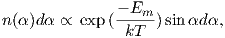(A.1)

where Em is the magnetic energy. When we measure the induced magnetization, we really measure only the component of the moment parallel to the applied field, or n(α)mcosα. The net induced magnetization MI of a population of particles with volume v is therefore: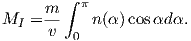(A.2)

By definition, n(α) integrates to N, the total number of moments, or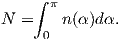(A.3)

The total saturation moment of a given population of N individual magnetic moments m is Nm. The saturation value of magnetization Ms is thus Nm normalized by the volume v. Therefore, the magnetization expressed as the fraction of saturation is: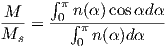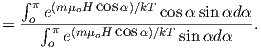By substituting a = oH∕kT and cosα = x, we write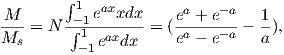(A.4)

and finally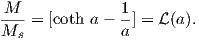(A.5)

#### A.2.2 Superparamagnetism

The derivation of superparamagnetism follows closely that of paramagnetism whereby the probability of finding a magnetization vector an angle α away from the direction of the applied field is give by: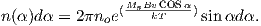(A.6)

The total magnetization contributed by the N moments is: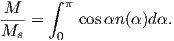(A.7)

Combining EquationsA.6) and  A.7 we get: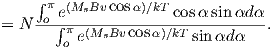By substituting a = MsBv∕kT and cosα = x, and remembering Equation A.5, we can write: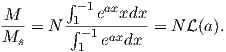(A.8)

So finally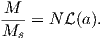(A.9)

### A.3 Useful tricks

In this section, we have assembled assorted mathematical and plotting techniques that come in handy through out this book.

#### A.3.1 Spherical trigonometry

Spherical trigonometry has widespread applications throughout the book. It is used in the transformations of observed directions to virtual poles (Chapter 2) and transformation of coordinate sytems, to name a few. Here we summarize the two most useful relationships: the Law of Sines and the Law of Cosines.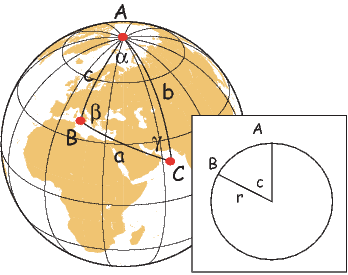Figure A.1: Rules of spherical trigonometry. a,b,c are all great circle tracks on a sphere which form a triangle with apices A,B,C. The lengths of a,b,c on a unit sphere are equal to the angles subtended by radii that intersect the globe at the apices, as shown in the inset. α,β,γ are the angles between the great circles.

In Figure A.1, α,β and γ are the angles between the great circles labelled a, b, and c. On a unit sphere, a,b and c are also the angles subtended by radii that intersect the globe at the apices A, B, and C (see inset on Figure A.1). Two formulae from spherical trigonometry come in handy in paleomagnetism, the Law of Sines: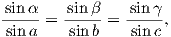(A.10)

and the Law of Cosines: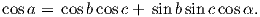(A.11)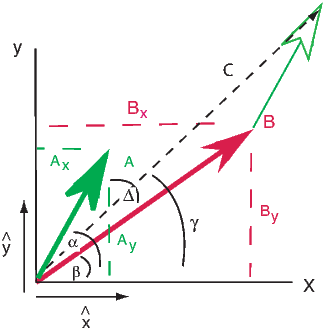Figure A.2: Vectors A and B, their components Ax,y, Bx,y and the angles between them and the X axis, α and β. The angle between the two vectors is α -β = Δ. Unit vectors in the directions of the axes are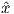and ŷ respectively.

To add the two vectors (see Figure A.2) A and B, we break each vector into components Ax,y and Bx,y. For example, Ax = |A|cosα,Ay = |A|sinα where |A| is the length of the vector A. The components of the resultant vector C are: Cx = Ax + Bx,Cy = Ay + By. These can be converted back to polar coordinates of magnitude and angles if desired, whereby: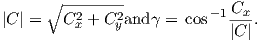#### A.3.3 Vector subtraction

To subtract two vectors, compute the components as in addition, but the components of the vector difference C are: Cx = Ax - Bx,Cy = Ay - By.

#### A.3.4 Vector multiplication

There are two ways to multiply vectors. The first is the dot product whereby A B = AxBx + AyBy. This is a scalar and is actually the cosine of the angle between the two vectors if the A and B are taken as unit vectors (assume a magnitude of unity in the component calculation.Figure A.3: Illustration of cross product of vectors A and B separated by angle θ to get the orthogonal vector C.

The other way to perform vector multiplication is the cross product (see Figure A.3), which produces a vector orthogonal to both A and B and whose components are given by:To calculate the determinant, we follow these rules: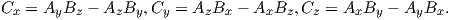or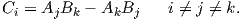#### A.3.5 Tricks with tensors

Vectors belong to a more general concept called tensors. While a vector describes a magnitude of something in a given direction, tensors allow calculation of magnitudes as a function of orientation. Velocity is a vector relating speed to direction, but speed may change depending on direction, so we might need a tensor to calculate speed as a function of direction. Many properties in Earth science require tensors, like the indicatrix in mineralogy which relates the speed of light to crystallographic direction, or the relationship between stress and strain. Tensors in paleomagnetism are used, for example, to transform coordinate systems and to characterize the anisotropy of magnetic properties such as susceptibility. We will cover transformation of coordinate systems in the following.

##### Direction cosines

We use direction cosines in paleomagnetism in a variety of applications, from mineralogy to transformation from specimen to geographic or stratigraphic coordinate systems. Direction cosines are the cosines of the angles between different axes in given coordinate systems, here X and Xrespectively (see, e.g., Figure A.4a.) The direction cosine a12 is the cosine of the angle between the X1 and the X2, α12 axes. We can define four of these direction cosines to fully describe the relationship between the two coordinate systems: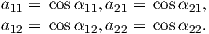The first subscript always refers to the X system and the second refers to the X.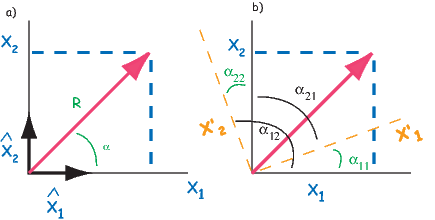Figure A.4: Definition of direction cosines in two dimensions. a) Definition of vector in one set of coordinates, x1,x2. b) Definition of angles relating X axes to X.

##### Changing coordinate systems

One application of using direction cosines is the transformation of coordinates systems from one set (X) to a new set X. To find new coordinates x1,x2,.. from the old (x1,x2,...), we have:In three dimensions we have: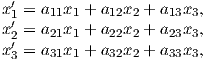which can also be written as: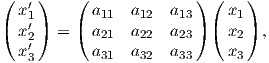(A.12)

with a short cut notation as: xi = aijxj. However we write this, it means that for each axis i, just sum through the j’s for all the dimensions. The matrix aij is an example of a 3 x 3 tensor and equations of the form Ai = BijCj relating two vectors with a tensor will be used throughout the book. A more common notation is with bold-faced variables which indicate vectors or tensors, e.g., A = B C.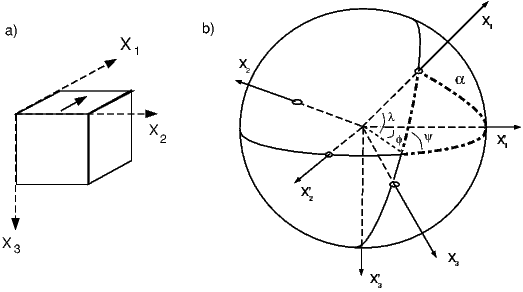Figure A.5: a) Sample coordinate system. b) Trigonometric relations between two cartesian coordinate systems, Xi and Xi. λ,ϕ,ψ are all known and the angles between the various axes can be calculated using spherical trigonometry. For example, the angle α between X1 and X1forms one side of the triangle shown by dash-dot lines. Thus, cosα = cosλcosϕ + sinλsinϕcosψ. [Figure from Tauxe, 1998.]

Now we would like to apply this to changing coordinate systems for a paleomagnetic specimen in the most general case. The specimen coordinate system is defined by a right-hand rule where the thumb (X1) is directed parallel to an arrow marked on the sample, the index finger (X2) is in the same plane but at right angles and clockwise to X1 and the middle finger (X3) is perpendicular to the other two (Figure A.5a). The transformation of coordinates (xi) from the Xi axes to the coordinates in the desired Xcoordinate system requires the determination of the direction cosines as described in Appendix A.3.5. The various aij can be calculated using spherical trigonometry as in Appendix A.3.1. For example, a11 for the general case depicted in Figure A.5 is cosα, which is given by the Law of Cosines (see Appendix A.3.1) by using appropriate values, or: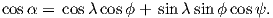The other aij can be calculated in a similar manner. In the case of most coordinate system rotations used in paleomagnetism, X2 is in the same plane as X1 and X2 (and is horizontal) so ψ = 90. This problem is much simpler. The directions cosines for the case where ψ = 90 are: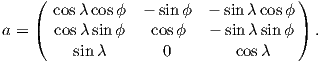(A.13)

The new coordinates can be obtained from Equation A.12, as follows: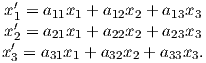(A.14)

The declination and inclination can be calculated by inserting these values in the equations in Chapter 2.

##### Method for rotating points on a globe using finite rotation poles

Given the coordinates of the point on the globe Pp with latitude λp, longitude ϕp the finite rotation pole Pf with latitude λf, longitude ϕf, the way to transform coordinates is as follows (you should also review Appendix A.3.5).

1. Convert the latitudes and longitudes to cartesian coordinates by: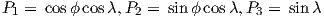where P is the point of interest.
2. Set up the rotation matrix R as:3. The coordinates of the transformed pole (Pt) are:which can be converted back into latitude and longitude in the usual way (see Chapter 2).

Table A.4: Finite rotations for selected Gondwana continents. Rotates continent to South African fixed coordinates. AUS: Australia, ANT: East Antarctica; IND: India; SAM: South American Craton. [Rotations of Torsvik et al. (2008) - see for additional data.]
 Age AUS ANT IND SAM Ma λ ϕ Ω λ ϕ Ω λ ϕ Ω λ ϕ Ω 5 9.7 54.3 -3.3 8.2 -49.4 0.8 22.7 32.9 -2.3 62.1 -40.2 1.6 10 10.4 52.8 -6.2 8.2 -49.4 1.5 23.8 33.1 -4.6 61.8 -40.3 3.3 15 11.5 49.8 -9 9.8 -48.4 2.1 27.1 27.4 -6 59.6 -38.1 5.4 20 12.4 48 -11.8 10.7 -47.9 2.8 29.6 23.9 -7.5 58.5 -37.1 7.5 25 12.9 48.3 -15 11.4 -48.2 3.8 25.1 33.2 -10.3 57.7 -36.4 9.6 30 12.8 49.9 -18.1 11.8 -48.3 4.8 22.5 38.5 -13.3 56.7 -34.5 11.9 35 13.5 50.8 -20.9 12.5 -46.1 6 22.6 41.3 -15.9 56.5 -33.4 14.3 40 14.1 52.7 -22.1 13.6 -41.5 7.4 25.5 42.7 -17.4 57.1 -32.6 16.6 45 14.4 54.7 -22.9 11.1 -41.1 8.5 24.2 40.1 -19.7 57 -31.4 18.6 50 14.7 56.5 -23.6 9.1 -40.9 9.6 24 34.2 -23.5 58.2 -31.2 20.5 55 14 57.3 -24.7 9.4 -43.5 10.3 22.1 29.2 -28.3 60.7 -31.9 22 60 12.9 57.9 -25.7 10.6 -47.4 10.8 19.5 25.2 -34.4 62.5 -32.8 23.3 65 13.6 58.8 -26.3 8.1 -47.7 11.3 19 21.9 -40.2 63.7 -33.5 24.6 70 17.3 60.2 -26.3 0.4 -43.3 12.2 20.5 18.9 -44.4 63.5 -33.4 26.1 75 19.8 63.3 -26.7 3.7 138.9 -13.8 21.8 18.2 -47.3 63.2 -33.9 28.6 80 20.5 68.5 -26.6 2.7 142.7 -16.1 22.3 18.2 -49.1 62.7 -34.3 31.5 85 19.8 74.6 -26.9 0.6 144.7 -18.8 21.8 22.1 -53.8 61.2 -34.3 34.4 90 17.7 80.9 -28.9 1.4 -37 22.3 20 27.5 -58.8 59.1 -34.5 37.3 95 15.9 86.2 -31.1 2.9 -38.3 25.8 20.7 28.1 -57.8 57.2 -34.7 40.3 100 18.4 89.3 -30.7 3.1 146.5 -26.8 21.3 28.8 -56.8 55.7 -34.8 43.3 105 17.9 95.6 -32.6 5.5 148.9 -30.3 21.9 29.6 -55.9 54.3 -34.9 46.4 110 17.3 101 -34.8 7.4 150.7 -33.9 22.6 30.3 -54.9 53.1 -35 49.5 115 16.8 105.6 -37.4 9 152.3 -37.6 23.3 31.1 -54 52.2 -35 51.7 120 16.4 109.4 -40.3 10.3 153.6 -41.3 24 32 -53.1 51.6 -35 52.8 125 15.7 110.3 -42.3 9.4 152.4 -43 23.4 34.8 -55.2 50.7 -33.9 54 130 15.9 111.6 -44.4 9.1 151.5 -45.3 21.2 36.2 -60.1 50.1 -32.8 54.9 135 15.9 113.1 -46.6 8.6 150.9 -47.6 21.2 36.2 -61.6 50 -32.5 55.1 140 15.6 113.7 -48.3 8 150.1 -49.2 21.9 37.5 -61.5 50 -32.5 55.1 145 15 113.1 -50.5 7.3 148.1 -50.7 22.6 39 -62.5 50 -32.5 55.1 150 15.5 113.5 -52.5 7.4 147.1 -52.6 24.1 40.4 -62.9 50 -32.5 55.1 155 17.6 115.7 -54.3 9 148 -55.4 26.9 41.2 -61.6 50 -32.5 55.1 160 19.5 117.8 -56.2 10.5 148.8 -58.2 29.8 42.1 -60.5 50 -32.5 55.1 165 19.5 117.8 -56.2 10.5 148.8 -58.2 29.8 42.1 -60.5 50 -32.5 55.1 170 19.5 117.8 -56.2 10.5 148.8 -58.2 29.8 42.1 -60.5 50 -32.5 55.1 175 19.5 117.8 -56.2 10.5 148.8 -58.2 29.8 42.1 -60.5 50 -32.5 55.1 180 19.5 117.8 -56.2 10.5 148.8 -58.2 29.8 42.1 -60.5 50 -32.5 55.1 185 19.5 117.8 -56.2 10.5 148.8 -58.2 29.8 42.1 -60.5 50 -32.5 55.1 190 19.5 117.8 -56.2 10.5 148.8 -58.2 29.8 42.1 -60.5 50 -32.5 55.1 195 19.5 117.8 -56.2 10.5 148.8 -58.2 29.8 42.1 -60.5 50 -32.5 55.1 200 19.5 117.8 -56.2 10.5 148.8 -58.2 29.8 42.1 -60.5 50 -32.5 55.1 205 19.5 117.8 -56.2 10.5 148.8 -58.2 29.8 42.1 -60.5 50 -32.5 55.1 210 19.5 117.8 -56.2 10.5 148.8 -58.2 29.8 42.1 -60.5 50 -32.5 55.1 215 19.5 117.8 -56.2 10.5 148.8 -58.2 29.8 42.1 -60.5 50 -32.5 55.1 220 19.5 117.8 -56.2 10.5 148.8 -58.2 29.8 42.1 -60.5 50 -32.5 55.1 225 19.5 117.8 -56.2 10.5 148.8 -58.2 29.8 42.1 -60.5 50 -32.5 55.1 230 19.5 117.8 -56.2 10.5 148.8 -58.2 29.8 42.1 -60.5 50 -32.5 55.1 235 19.5 117.8 -56.2 10.5 148.8 -58.2 29.8 42.1 -60.5 50 -32.5 55.1 240 19.5 117.8 -56.2 10.5 148.8 -58.2 29.8 42.1 -60.5 50 -32.5 55.1 245 19.5 117.8 -56.2 10.5 148.8 -58.2 29.8 42.1 -60.5 50 -32.5 55.1 250 19.5 117.8 -56.2 10.5 148.8 -58.2 29.8 42.1 -60.5 50 -32.5 55.1 255 19.5 117.8 -56.2 10.5 148.8 -58.2 29.8 42.1 -60.5 50 -32.5 55.1 260 19.5 117.8 -56.2 10.5 148.8 -58.2 29.8 42.1 -60.5 50 -32.5 55.1 265 19.5 117.8 -56.2 10.5 148.8 -58.2 29.8 42.1 -60.5 50 -32.5 55.1 270 19.5 117.8 -56.2 10.5 148.8 -58.2 29.8 42.1 -60.5 50 -32.5 55.1 275 19.5 117.8 -56.2 10.5 148.8 -58.2 29.8 42.1 -60.5 50 -32.5 55.1 280 19.5 117.8 -56.2 10.4 148.8 -58.2 29.8 42.1 -60.5 50 -32.5 55.1 285 19.5 117.8 -56.2 10.5 148.8 -58.2 29.8 42.1 -60.5 50 -32.5 55.1 290 19.5 117.8 -56.2 10.5 148.8 -58.2 29.8 42.1 -60.5 50 -32.5 55.1 295 19.5 117.8 -56.2 10.5 148.8 -58.2 29.8 42.1 -60.5 50 -32.5 55.1 300 19.5 117.8 -56.2 10.5 148.8 -58.2 29.8 42.1 -60.5 50 -32.5 55.1 305 19.5 117.8 -56.2 10.4 148.8 -58.2 29.8 42.1 -60.5 50 -32.5 55.1 310 19.5 117.8 -56.2 10.5 148.8 -58.2 29.8 42.1 -60.5 50 -32.5 55.1 315 19.5 117.8 -56.2 10.5 148.8 -58.2 29.8 42.1 -60.5 50 -32.5 55.1 320 19.5 117.8 -56.2 10.5 148.8 -58.2 29.8 42.1 -60.5 50 -32.5 55.1

Table A.5: Finite rotations for selected Laurentian continents. Rotates continent to South African fixed coordinates. EUR: Europe; NAM: North America; GRN: Greenland. [Rotations of Torsvik et al. (2008) - see for additional data.]
 Age EUR NAM GRN Ma λ ϕ Ω λ ϕ Ω λ ϕ Ω EUR NAM GRN 5 17.9 -27.1 0.6 80.9 22.8 1.3 80.9 22.8 1.3 10 18.4 -26.3 1.2 80.9 22.9 2.6 80.9 22.9 2.6 15 18.9 -24.6 1.8 80.9 23.2 4.1 80.9 23.2 4.1 20 17.2 -22.7 2.4 80.6 24.4 5.5 80.6 24.4 5.5 25 20.7 -19 3 79.5 28.1 6.8 79.5 28.1 6.8 30 24.9 -19.5 4.3 77.3 12.5 8.6 77.3 12.5 8.6 35 27.2 -19.3 5.8 75.4 3.5 10.5 74.8 7.2 10.2 40 28.7 -18.5 7.5 74.5 -1.1 12.6 72.6 9.5 11.5 45 30.3 -18.2 9 74.3 -4.3 14.6 71.4 11.4 12.7 50 30.8 -16.7 10 75.9 -3.5 16.2 71 20.7 14.2 55 32.7 -15.4 11.3 79.8 4.1 17.6 71.8 29.6 16.8 60 34.8 -15.7 12.6 81.6 5.1 19.1 71.9 30.5 17.5 65 36 -15.8 13.6 82.6 3.2 20.7 71.3 32.9 17.6 70 35.4 -16.1 14.9 81.6 -6.5 22.4 69.8 29 17.9 75 35.5 -15.7 15.5 80.4 -13.1 24.6 69 26.6 18.5 80 36.1 -15.2 16.9 78.2 -18.8 27.5 67.6 21 19.8 85 37 -14.2 18.8 76.2 -21.3 30.5 66.3 16.4 21.5 90 39.6 -13.7 21.9 74.6 -23 33.8 65.9 11.5 24.2 95 39.8 -13.7 25.2 72 -24.7 36.9 64.2 5.5 26.9 100 40.2 -12.5 28.5 70 -24 40.2 62.7 2.8 30.1 105 41.6 -11.2 31.7 69.1 -23.3 43.6 62.4 1.6 33.3 110 42.6 -9.8 34.5 68.3 -22.6 47 62.1 0.9 36.5 115 43.4 -8.5 37.3 67.6 -21.8 50.4 61.8 0.5 39.7 120 44.5 -6.9 40.3 67.1 -20.4 53.9 61.8 0.8 43.1 125 45.3 -6.3 42 67 -19.7 55.6 61.9 1 44.9 130 45.9 -5.7 43 67 -19.1 56.7 62.2 1.3 46 135 46.6 -5.3 44 67.1 -18.7 57.9 62.4 1.6 47.1 140 47.3 -4.9 45.2 67.2 -18.4 59.2 62.7 1.6 48.4 145 47.8 -4.8 46.4 67.1 -18.3 60.5 62.9 1.3 49.7 150 48.6 -4 47.9 67.3 -17.6 62.2 63.2 1.8 51.4 155 49.8 -2.2 50 67.6 -15.5 64.6 63.7 3.6 53.8 160 50.6 -1.2 52.1 67.6 -14.5 66.8 64.1 4.2 56 165 51.4 -0.3 54.2 67.7 -13.6 69.1 64.4 4.8 58.3 170 52.1 0.6 56.3 67.8 -12.8 71.4 64.7 5.3 60.6 175 52.9 1.9 59.6 67.7 -11.5 74.8 64.8 6 64.1 180 53 2 60 67.7 -11.5 75.3 64.9 6 64.5 185 53 2 60.4 67.7 -11.5 75.7 64.9 5.9 64.9 190 53.1 2.1 60.8 67.7 -11.5 76.1 65 5.9 65.4 195 53.2 2.2 61.1 67.7 -11.5 76.6 65 5.8 65.8 200 53.3 2.2 61.5 67.7 -11.5 77 65.1 5.8 66.2 205 53.2 2.6 59.7 67.7 -11.5 77.4 65.1 5.7 66.7 210 53.1 2.9 57.8 67.7 -11.5 77.9 65.2 5.7 67.1 215 53.1 3.3 55.9 67.7 -11.5 78.3 65.2 5.6 67.5 220 52.9 3.6 53.6 67.7 -11.5 78.3 65.2 5.6 67.5 225 52.7 4 51.4 67.7 -11.5 78.3 65.2 5.6 67.5 230 52.4 4.4 49.1 67.7 -11.5 78.3 65.2 5.6 67.5 235 52.2 4.8 46.8 67.7 -11.5 78.3 65.2 5.6 67.5 240 51.9 5.3 44.5 67.7 -11.5 78.3 65.2 5.6 67.5 245 51.9 5.3 44.5 67.7 -11.5 78.3 65.2 5.6 67.5 250 51.9 5.3 44.5 67.7 -11.5 78.3 65.2 5.6 67.5 255 51.9 5.3 44.5 67.7 -11.5 78.3 65.2 5.6 67.5 260 51.9 5.3 44.5 67.7 -11.5 78.3 65.2 5.6 67.5 265 51.9 5.3 44.5 67.7 -11.5 78.3 65.2 5.6 67.5 270 51.9 5.3 44.5 67.7 -11.5 78.3 65.2 5.6 67.5 275 51.9 5.3 44.5 67.7 -11.5 78.3 65.2 5.6 67.5 280 51.9 5.3 44.5 67.7 -11.5 78.3 65.2 5.6 67.5 285 51.9 5.3 44.5 67.7 -11.5 78.3 65.2 5.6 67.5 290 51.9 5.3 44.5 67.7 -11.5 78.3 65.2 5.6 67.5 295 51.9 5.3 44.5 67.7 -11.5 78.3 65.2 5.6 67.5 300 51.9 5.3 44.5 67.7 -11.5 78.3 65.2 5.6 67.5 305 51.9 5.3 44.5 67.7 -11.5 78.3 65.2 5.6 67.5 310 51.9 5.3 44.5 67.7 -11.5 78.3 65.2 5.6 67.5 315 51.9 5.3 44.5 67.7 -11.5 78.3 65.2 5.6 67.5 320 51.9 5.3 44.5 67.7 -11.5 78.3 65.2 5.6 67.5

Table A.6: Finite rotations for South Africa to the paleomagnetic reference frame. All rotation pole latitudes are assumed to be zero. Ages are in Ma and rotation angles are in degree.[Rotations of Torsvik et al. (2008) - see for additional options.]
 Age ϕ Ω Age ϕ Ω Age ϕ Ω Age ϕ Ω 5 56 2.2 85 138.1 19.3 165 157 30.7 245 137.4 36.5 10 57.6 2.5 90 142.9 19.6 170 159.5 32.5 250 143.1 39.6 15 53.9 2.5 95 144.7 20.5 175 167.6 28.8 255 145.4 40.4 20 66.5 3 100 144.3 20.8 180 167.8 27.7 260 145.6 41.8 25 75.5 4.7 105 150.8 22.3 185 167.4 25.9 265 144.8 41.9 30 84.1 6.8 110 160.2 26.9 190 168.4 21.6 270 141.6 47.1 35 95.8 7.9 115 169.2 32.1 195 158.8 18.2 275 140.3 46.8 40 98.8 8.7 120 170.3 35.6 200 147.9 17.8 280 138.2 51.1 45 107.5 9.2 125 171.3 36.2 205 144.4 19.2 285 138.6 51.6 50 110.9 10.3 130 172.1 37.5 210 137.4 20.7 290 136.5 51.8 55 111.6 13.2 135 170 39.4 215 133.6 23.1 295 135.8 52.8 60 115.7 13.9 140 172.6 42.1 220 129.9 26.4 300 136.8 53.5 65 123.5 15.7 145 163.1 40.8 225 127.2 27.2 305 136.9 55.4 70 127.8 17.5 150 155.2 38.1 230 128 29.4 310 138.9 56.3 75 137.2 17.5 155 155 34.8 235 130 31.4 315 139.9 59.5 80 140.3 19.2 160 155 33.2 240 133.6 35.3 320 138.9 60.8

##### The orientation tensor and eigenvectors

The orientation tensor T (Scheidegger, 1965) (also known as the matrix of sums of squares and products), is extremely useful in paleomagnetism. This is found as follows:

1. Convert the D, I, and M for a set of data points (e.g., a sequence of demagnetization data, or a set of geomagnetic vectors or unit vectors where M = 1) to corresponding xi values (see Chapter 2).
2. Calculate the coordinates of the “center of mass” (x) of the data points: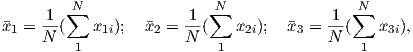(A.15)

where N is the number of data points involved. Note that for unit vectors, the center of mass is the same as the Fisher mean (Chapter 11).

3. Transform the origin of the data cluster to the center of mass:(A.16)

where xi are the transformed coordinates.

4. The orientation matrix is defined as: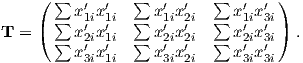(A.17)

T is a 3 x 3 matrix, where only six of the nine elements are independent. It is constructed in some coordinate system, such as the geographic or sample coordinate system. Usually, none of the six independent elements are zero. There exists, however, a coordinate system along which the “off-axis” terms are zero and the axes of this coordinate system are called the eigenvectors of the matrix. The three elements of T in the eigenvector coordinate system are called eigenvalues. In terms of linear algebra, this idea can be expressed as: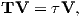(A.18)

where V is the matrix containing three eigenvectors and τ is the diagonal matrix containing three eigenvalues. Equation A.18 is only true if: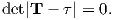(A.19)

If we expand equation A.19, we have a third degree polynomial whose roots (τ) are the eigenvalues: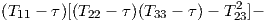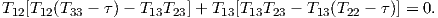The three possible values of τ (τ123) can be found with iteration and determination. In practice, there are many programs for calculating τ. My personal favorite is the Numpy Module for Python (see many free websites, especially Scientific Python (SciPy) for hints). Please note that the conventions adopted here are to scale the τ’s such that they sum to one; the largest eigenvalue is termed τ1 and corresponds to the eigenvector V1.

Inserting the values for the transformed components calculated in equation  A.16 into T gives the covariance matrix for the demagnetization data. The direction of the axis associated with the greatest scatter in the data (the principal eigenvector V1) corresponds to a best-fit line through the data. This is usually taken to be the direction of the component in question. This direction also corresponds to the axis around which the “moment of inertia” is least. The eigenvalues of T are the variances associated with each eigenvector. Thus the standard deviations are σi =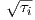.

#### A.3.6 Upside down triangles, ∇

We often wish to differentiate a function along three orthogonal axes. For example, imagine we know the topography of a ski area (see Figure A.6). For every location (in say, X and Y coordinates), we know the height above sea level. This is a scalar function. Now imagine we want to build a ski resort, so we need to know the direction of steepest descent and the slope (red arrows in Figure A.6).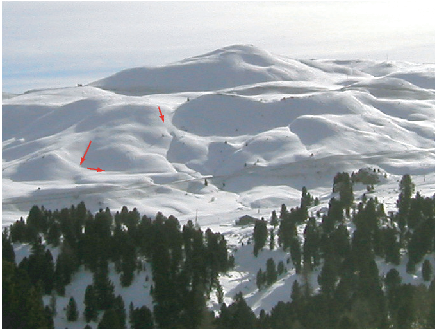Figure A.6: Illustration of the relationship between a vector field (direction and magnitude of steepest slope at every point, e.g., red arrows) and the scalar field (height) of a ski slope.

To convert the scalar field (height versus position) to a vector field (direction and magnitude of greatest slope) mathematically, we would simply differentiate the topography function. Let’s say we had a very weird two dimensional, sinusoidal topography such that z = f(x) = sinx with z the height and x is the distance from some marker. The slope in the x direction (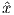), then would be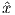d _ dxf(x). If f(x,y,z) were a three dimentional topography then the gradient of the topography function would be: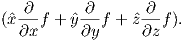For short hand, we define a “vector differential operator” to be a vector whose components are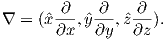This can also be written in polar coordinates: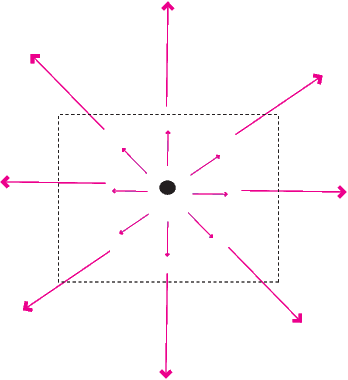Figure A.7: Example of a vector field with a non-zero divergence.

##### Divergence

The divergence of a vector function (e.g. H) is written as: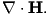The trick here is to treat as a vector and use the rules for dot products described in Appendix A.3.2. In cartesian coordinates, this is: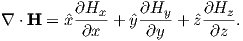Like all dot products, the divergence of a vector function is a scalar.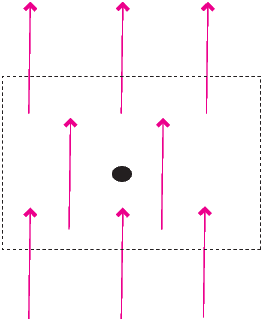Figure A.8: Example of a vector field with zero divergence.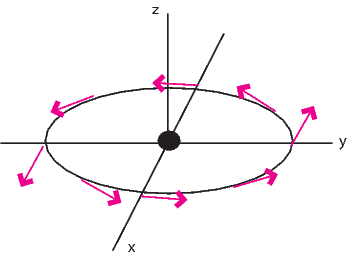Figure A.9: Example of a vector field with non-zero curl.

The name divergence is well chosen because ∇⋅ H is a measure of how much the vector field “spreads out” (diverges) from the point in question. In fact, what divergence quantifies is the balance between vectors coming in to a particular region versus those that go out. The example in Figure A.7 depicts a vector function whereby the magnitude of the vector increases linearly with distance away from the central point. An example of such a function would be v(r) = r. The divergence of this function is:(a scalar). There are no arrows returning in to the dashed box, only vectors going out and the non-zero divergence quantifies this net flux out of the box.

Now consider Figure A.8, which depicts a vector function that is constant over space, i.e. v(r) = k. The divergence of this function is: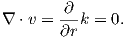The zero divergence means that for every vector leaving the box, there is an equal and opposite vector coming in. Put another way, no net flux results in a zero divergence. The fact that the divergence of the magnetic field is zero means that there are no point sources (monopoles), as opposed to electrical fields that have divergence related to the presence of electrons or protons.

##### Curl

The curl of the vector function B is defined as ∇× B. In cartesian coordinates we have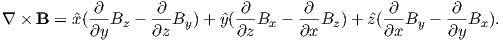Curl is a measure of how much the vector function “curls” around a given point. The function describing the velocity of water in a whirlpool has a significant curl, while that of a smoothly flowing stream does not.

Consider Figure A.9 which depicts a vector function v = -y+ xŷ. The curl of this function is: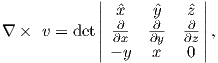or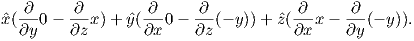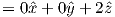So there is a positive curl in this function and the curl is a vector in the direction.

The magnetic field has a non-zero curl in the presence of currents or changing electric fields. In free space, away from currents (lightning!!), the magnetic field has zero curl.

#### A.3.7 The statistical bootstrap

Sometimes things just are not normal. Statistically that is. When you can not assume that your data follow some known distribution, like the normal distribution, or the Fisher distribution, what do you do? In this section, we outline a technique called the bootstrap, which allows us to make statistical inferences when parametric assumptions fail. The reader should also refer to Efron and Tibshirani (1993) for a more complete discussion.

In Figure A.10, we illustrate the essentials of the statistical bootstrap. We will develop the technique using data drawn from a normal distribution. First, we generate a synthetic data set by drawing 500 data points from a normal distribution with a mean x of 10 and a standard deviation σ of 2. The synthetic data are plotted as a histogram in Figure A.10a. In Figure A.10b we plot the data as a Q-Q plot (see Appendix B.1.5) against the zi expected for a normal distribution.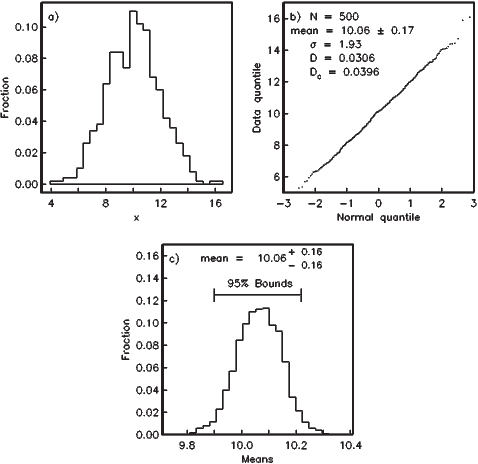Figure A.10: Bootstrapping applied to a normal distribution. a) 500 data points are drawn from a Gaussian distribution with mean of 10 and a standard deviation of 2. b) Q-Q plot of data in a). The 95% confidence interval for the mean is given by Gauss statistics as 0.17. 10,000 new (para) data sets are generated by randomly drawing N data points from the original data set shown in a). c) A histogram of the means from all the para-data sets. 95% of the means fall within the interval 10.06-0.16+0.16, hence the bootstrap confidence interval is similar to that calculated with Gaussian statistics. [Figure from Tauxe, 1998.]

The data in Figure A.10a plot in a line on the Q-Q plot (Figure A.10b). The value for D is 0.0306. Because N = 500, the critical value of D, Dc at the 95% confidence level is 0.0396. Happily, our normal distribution simulation program has produced a set of 500 numbers for which the null hypothesis of a normal distribution has not been rejected. The mean of the synthetic dataset is about 10 and the standard deviation is 1.9. The usual Gaussian statistics allow us to estimate a 95% confidence interval for the mean as 1.96σ∕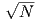or 0.17.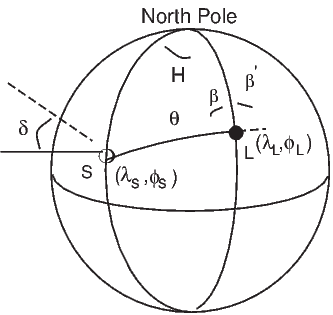Figure A.11: Calculation of the azimuth of the shadow direction (β) relative to true North, using a sun compass. L is the site location (at λLL), S is the position on the Earth where the sun is directly overhead (λSS). [Figure from Tauxe, 1998.]

In order to estimate a confidence interval for the mean using the bootstrap, we first randomly draw a list of N data by selecting data points from the original data set. This list is called a pseudo-sample of the data. Some data points will be used more than once and others will not be used at all. We then calculate the mean of the pseudo-sample. We repeat the procedure of drawing pseudo-samples and calculating the mean many times (say 10,000 times). A histogram of the “bootstrapped” means is plotted in Figure A.10c. If these are sorted such that the first mean is the lowest and the last mean is the highest, the 95% of the means are between the 250th and the 9,750th mean. These therefore are the 95% confidence bounds because we are approximately 95% confident that the true mean lies between these limits. The 95% confidence interval calculated for the data in Figure A.10 by bootstrap is about 0.16 which is nearly the same as that calculated the Gaussian way. However, the bootstrap required orders of magnitude more calculations than the Gaussian method, hence it is ill-advised to perform a bootstrap calculation when a parametric one will do. Nonetheless, if the data are not Gaussian, the bootstrap provides a means of calculating confidence intervals when there is no quick and easy way. Furthermore, with a modern computer, the time required to calculate the bootstrap illustrated in Figure A.10 was virtually imperceptible.

#### A.3.8 Directions using a sun compass

In a sun compass problem, we have the direction of the sun’s shadow and an angle between that and the desired direction (α). The declination of the shadow itself is 180 from the direction toward the sun. In Figure A.11, the problem of calculating declination from sun compass information is set up as a spherical trigonometry problem, similar to those introduced in Chapter 2 and Appendix A.3.1. The declination of the shadow direction β, is given by 180 - β. We also know the latitude of the sampling location L (λL). We need to calculate the latitude of S (the point on the Earth’s surface where the sun is directly overhead), and the local hour angle H.

Knowing the time of observation (in Universal Time), the position of S (λs = δ,ϕs in Figure A.11) can be calculated with reasonable precision (to within 0.01) for the period of time between 1950 and 2050 using the procedure recommended in the 1996 Astronomical Almanac:

1. First, calculate the Julian Day J. Then, calculate the fraction of the day in Universal Time U. Finally, calculate the parameter d which is the number of days from J2000 by: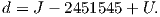2. The mean longitude of the sun (ϕs), corrected for aberration, can be estimated in degrees by: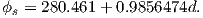3. The mean anomaly g = 357.528 + 0.9856003d (in degrees).
4. Put ϕs and g in the range 0 360.
5. The longitude of the ecliptic is given by ϕE = ϕs + 1.915sing + 0.020sin2g (in degrees).
6. The obliquity of the ecliptic is given by ϵ = 23.439 - 0.0000004d.
7. Calculate the right ascension (A) by:where f = 180∕π and t =tan2ϵ∕2.
8. The so-called “declination” of the sun (δ in Figure A.11 which should not be confused with the magnetic declination D), which we will use as the latitude λs, is given by: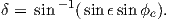9. Finally, the equation of time in degrees is given by E = 4(ϕs - A).

We can now calculate the Greenwich Hour Angle GHA from the Universal Time U (in minutes) by GHA = (U + E)4 + 180. The local hour angle (H in Figure A.11) is GHA + ϕL. We calculate β using the laws of spherical trigonometry (see Appendix A.3.1). First we calculate θ by the Law of Cosines (remembering that the cosine of the colatitude equals the sine of the latitude):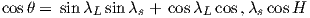and finally using the Law of Sines:If λs < λL, then the required angle is the shadow direction β, given by: β= 180 - β. The azimuth of the desired direction is βplus the measured shadow angle α.